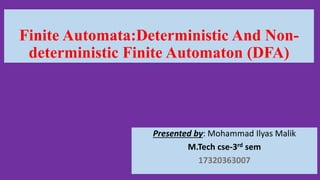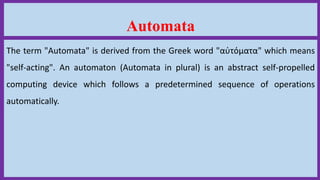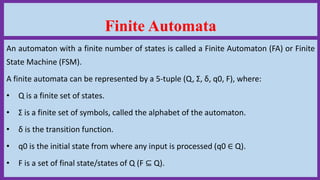Seu SlideShare está sendo baixado. ×

# Finite Automata: Deterministic And Non-deterministic Finite Automaton (DFA)

Anúncio
Anúncio
Anúncio
Anúncio
Anúncio
Anúncio
Anúncio
Anúncio
Anúncio
Anúncio
AnúncioCarregando em…3
×

1 de 24 Anúncio

# Finite Automata: Deterministic And Non-deterministic Finite Automaton (DFA)

The term "Automata" is derived from the Greek word "αὐτόματα" which means "self-acting". An automaton (Automata in plural) is an abstract self-propelled computing device which follows a predetermined sequence of operations automatically.

The term "Automata" is derived from the Greek word "αὐτόματα" which means "self-acting". An automaton (Automata in plural) is an abstract self-propelled computing device which follows a predetermined sequence of operations automatically.

Anúncio
Anúncio

### Finite Automata: Deterministic And Non-deterministic Finite Automaton (DFA)

1. 1. Finite Automata:Deterministic And Non- deterministic Finite Automaton (DFA) Presented by: Mohammad Ilyas Malik M.Tech cse-3rd sem 17320363007
2. 2. Automata The term "Automata" is derived from the Greek word "αὐτόματα" which means "self-acting". An automaton (Automata in plural) is an abstract self-propelled computing device which follows a predetermined sequence of operations automatically.
3. 3. Finite Automata An automaton with a finite number of states is called a Finite Automaton (FA) or Finite State Machine (FSM). A finite automata can be represented by a 5-tuple (Q, Σ, δ, q0, F), where: • Q is a finite set of states. • Σ is a finite set of symbols, called the alphabet of the automaton. • δ is the transition function. • q0 is the initial state from where any input is processed (q0 ∈ Q). • F is a set of final state/states of Q (F ⊆ Q).
4. 4. Deterministic Finite Automaton (DFA) In DFA, for each input symbol, one can determine the state to which the machine will move. Hence, it is called Deterministic Automaton. As it has a finite number of states, the machine is called Deterministic Finite Machine or Deterministic Finite Automaton.
5. 5. A DFA can be represented by a 5-tuple (Q, Σ, δ, q0, F) where: • Q is a finite set of states. • Σ is a finite set of symbols called the alphabet. • δ is the transition function where δ: Q × Σ → Q • q0 is the initial state from where any input is processed (q0 ∈ Q). • F is a set of final state/states of Q (F ⊆ Q).
6. 6. Graphical Representation of a DFA A DFA is represented by digraphs called state diagram. • The vertices/circles represent the states. • The arcs labeled with an input alphabet show the transitions. • The initial state is denoted by an empty single incoming arc. • The final state is indicated by double circles.
7. 7. Example Let a deterministic finite automaton be • Q = {a, b, c}, • Σ = {0, 1}, • q0={a}, • F={c}, and • Transition function δ as shown by the following table:
8. 8. Non-deterministic Finite Automaton In NDFA, for a particular input symbol, the machine can move to any combination of the states in the machine. In other words, the exact state to which the machine moves cannot be determined. Hence, it is called Non-deterministic Automaton. As it has finite number of states, the machine is called Non- deterministic Finite Machine or Nondeterministic Finite Automaton.
9. 9. An NDFA can be represented by a 5-tuple (Q, Σ, δ, q0, F) where: • Q is a finite set of states. • Σ is a finite set of symbols called the alphabets. • δ is the transition function where δ: Q × Σ → 2 𝑄 (Here the power set of Q (2 𝑄) has been taken because in case of NDFA, from a state, transition can occur to any combination of Q states) • q0 is the initial state from where any input is processed (q0 ∈ Q). • F is a set of final state/states of Q (F ⊆ Q).
10. 10. Graphical Representation of an NDFA Graphical Representation of an NDFA: (same as DFA) An NDFA is represented by digraphs called state diagram. • The vertices/Circles represent the states. • The arcs labeled with an input alphabet show the transitions. • The initial state is denoted by an empty single incoming arc. • The final state is indicated by double circles.
11. 11. Example Let a non-deterministic finite automaton be • Q = {a, b, c} • Σ = {0, 1} • q0 = {a} • F={c} and • Transition function δ as shown by the following table:
12. 12. DFA vs NDFA DFA NDFA The transition from a state is to a single particular next state for each input symbol. Hence it is called deterministic. The transition from a state can be to multiple next states for each input symbol. Hence it is called non-deterministic. Empty string transitions are not seen in DFA. NDFA permits empty string transitions. Backtracking is allowed in DFA In NDFA, backtracking is not always possible. Requires more space. Requires less space. A string is accepted by a DFA, if it transits to a final state. A string is accepted by a NDFA, if at least one of all possible transitions ends in a final state.
13. 13. NDFA to DFA Conversion Problem Statement Let X = (Qx, Σ, δx, q0, Fx) be an NDFA which accepts the language L(X). We have to design an equivalent DFA Y = (Qy, Σ, δy, q0, Fy) such that L(Y) = L(X). The following procedure converts the NDFA to its equivalent DFA:
14. 14. Algorithm Input: An NDFA Output: An equivalent DFA Step 1 Create state table from the given NDFA. Step 2 Create a blank state table under possible input alphabets for the equivalent DFA. Step 3 Mark the start state of the DFA by q0 (Same as the NDFA). Step 4 Find out the combination of States {Q0, Q1,... , Qn} for each possible input alphabet. Step 5 Each time we generate a new DFA state under the input alphabet columns, we have to apply step 4 again, otherwise go to step 6. Step 6 The states which contain any of the final states of the NDFA are the final states of the equivalent DFA.
15. 15. Example Let us consider the NDFA shown in the figure below.
16. 16. Using the above algorithm, we find its equivalent DFA. The state table of the DFA is shown in below.
17. 17. The state diagram of the DFA is as follows:
18. 18. Language Decidability A language is called Decidable or Recursive if there is a Turing machine which accepts and halts on every input string w. Every decidable language is Turing- Acceptable.
19. 19. A decision problem P is decidable if the language L of all yes instances to P is decidable. For a decidable language, for each input string, the TM halts either at the accept or the reject state as depicted in the following diagram:
20. 20. Example 1 Find out whether the following problem is decidable or not: Is a number ‘m’ prime? Solution Prime numbers = {2, 3, 5, 7, 11, 13, …………..} Divide the number ‘m’ by all the numbers between ‘2’ and ‘√m’ starting from ‘2’. If any of these numbers produce a remainder zero, then it goes to the “Rejected state”, otherwise it goes to the “Accepted state”. So, here the answer could be made by ‘Yes’ or ‘No’. Hence, it is a decidable problem.
21. 21. Example 2 Given a regular language L and string w, how can we check if w∈ L?